# (i) In the given figure, if AB || CD, find the value of x.

Question:

(i) In the given figure, if AB || CD, find the value of x.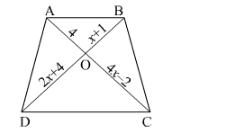(ii) In the given figure, If AB || CD, find the value of x.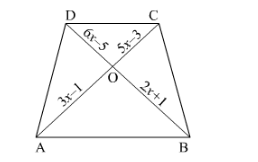(iii) In the given figure, AB || CD, If OA = 3x − 19, OB = x − 4, OC = x − 3 and OD = 4, find x.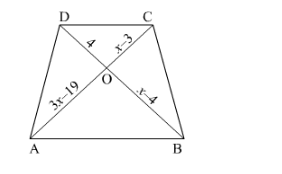Solution:

(i) It is given that $A B \| C D$.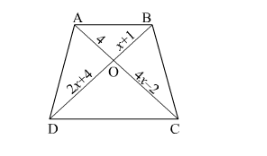We have to find the value of $x$.

Diagonals of the para

Now $\frac{D O}{O A}=\frac{C O}{O B}$

$\Rightarrow \frac{4 x-2}{4}=\frac{2 x+4}{x+1}$

$4(2 x+4)=(4 x-2)(x+1)$

$8 x+16=x(4 x-2)+1(4 x-2)$

$8 x+16=4 x^{2}-2 x+4 x-2$

$-4 x^{2}+8 x+16+2-2 x=0$

$-4 x^{2}+6 x+18=0$

$4 x^{2}-6 x-18=0$

$4 x^{2}-12 x+6 x-18=0$

So

$4 x(x-3)+6(x-3)=0$

$(4 x+6)(x-3)=0$

Therefore $4 x+6=0$ and $x-3=0$

Hence $x=3$

(ii) It is given that $A B \| C D$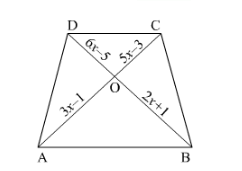We have to find the value of $x$

Now $\frac{D O}{O A}=\frac{C O}{O B}$

$\Rightarrow \frac{6 x-5}{2 x+1}=\frac{5 x-3}{3 x-1}$

$(6 x-5)(3 x-1)=(2 x+1)(5 x-3)$

$3 x(6 x-5)-1(6 x-5)=2 x(5 x-3)+1(5 x-3)$

$18 x^{2}-15 x-6 x+5=10 x^{2}-6 x+5 x-3$

$18 x^{2}-10 x^{2}-21 x+5+x+3=0$

$8 x^{2}-20 x+8=0$

$8 x^{2}-16 x-4 x+8=0$

$8 x(x-2)-4(x-2)=0$

$(8 x-4)(x-2)=0$

So

$(8 x-4)(x-2)=0$

Therefore $8 x-4=0$ or $x-2=0$

Hence x = 12 or x = 2.

For $x=12, \mathrm{OD}$ is negative.

Hence $x=2$

(iii) It is given that $A B \| C D$.

And $O A=3 x-19, O B=x-4 O C=x-3$ and $O D=4$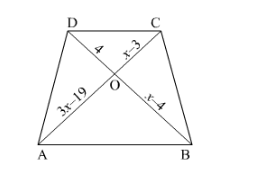We have to find the value of $x$

Now $\frac{A O}{O C}=\frac{B O}{O D}$

$\Rightarrow \frac{3 x-19}{x-3}=\frac{x-4}{4}$

$4(3 x-19)=(x-3)(x-4)$

$12 x-76=x(x-4)-3(x-4)$

$12 x-76=x^{2}-4 x-3 x+12$

$12 x-76=x^{2}-7 x+12$

$-x^{2}+7 x-12+12 x-76=0$

$-x^{2}+19 x-88=0$

$x^{2}-19 x+88=0$

So

$x^{2}-11 x-8 x+88=0$

$x(x-11)-8(x-11)=0$

Therefore $x-11=0$ and $x-8=0$

Hence $x=8$ or $x=11$.